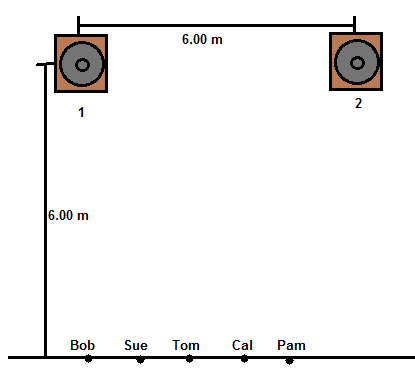# Two identical speakers (labeled 1 and 2) are playing a tone with a frequency of 155 Hz, in phase....

## Question:

Two identical speakers (labeled 1 and 2) are playing a tone with a frequency of {eq}155\ Hz {/eq}, in phase. The centers of the speakers are located 6.00 m apart. Five observers are standing on a line that is 6.00 m in front of the speakers as shown in the figure. Each observer is separated by 1.00 m and Bob is directly in front of speaker 1. Determine which observer or observers will experience constructive interference from the sound emitted by the speakers. The speed of sound is 343 m/s for this problem.## Interference:

Wave interference is a superposition of multiple waves. If the waves happen to align such that their peaks occur simultaneously, this is known as constructive interference. If two waves align such that the peak of one corresponds to the trough of the other, this is destructive interference. If the amplitudes of wave interfering destructively are also the same, then the superposition is the same as no wave at all, this is perfectly destructive interference.

The relative positions of the peaks and troughs are known as the phase difference. If two waves intefere constuctively, they are in phase; if they interfere destructively, they are {eq}180^\circ {/eq} out of phase.

Since the sounds are produced in phase, we can look at the travel of a single point of each wave generated at the same time. If these points reach the interference point at the same time, then the waves are still in phase there, and the interference is constructive. This occurs only along a single line which is equidistant from both. However, waves are repetitive, so if the the peak generated at one interval happens to align with the peak generated in another interval, the interference is still constructive. So, if we compare the distance of the interference point from both sources, and find that the difference in those distance is a whole number multiple of the wavelength, then the peaks will still overlap.

So, the condition that we have is:

{eq}r_1=r_2 + \lambda N {/eq}

Where r represents the distance of the point from the source, {eq}\lambda {/eq} is the wavelength of the sound, and N is an integer multiple (including negatives and zero).

The wavelength can be calculated from the speed and frequency by:

{eq}\lambda=\frac{v}{f}=\frac{343 \ \frac{m}{s}}{155 \ Hz}=2.21 \ m {/eq}

The distances for each person can be solve with some the Pythagorean Theorem, since we have the distance to the line of people, and the distance along the line each is at.

{eq}r_{Bob1}=6 \ m {/eq}

{eq}r_{Bob2}=\sqrt{6^2+6^2}=8.49 \ m {/eq}

{eq}r_{Sue1}=\sqrt{6^2+1^2}=6.08 \ m {/eq}

{eq}r_{Sue2}=\sqrt{6^2+5^2}=7.81 \ m {/eq}

{eq}r_{Tom1}=\sqrt{6^2+2^2}=6.32 \ m {/eq}

{eq}r_{Tom2}=\sqrt{6^2+4^2}=7.21 \ m {/eq}

{eq}r_{Pam1}=\sqrt{6^2+4^2}=7.21 \ m {/eq}

{eq}r_{Pam2}=\sqrt{6^2+2^2}=6.32 \ m {/eq}

Note we didn't calculate Cal's distances, this is because he is one that symmetry line between the two sources, and he will get constructive interference.

None of the other pairs are any whole number multiple of {eq}\lambda {/eq} from each other. So only Cal experiences constructive interference.